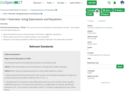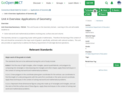Subject:
Mathematics
Material Type:
Full Course
Level:
Middle School
6
Provider:
CT State Department of Education
Provider Set:
CSDE - Public
Tags:
Language:
English
Media Formats:
Text/HTML

# Connecticut Model Math for Grade 6

Equitable and Inclusive Curriculum

The CSDE believes in providing a set of conditions where learners are repositioned at the center of curricula planning and design. Curricula, from a culturally responsive perspective, require intentional planning for diversity, equity, and inclusion in the development of units and implementation of lessons. It is critical to develop a learning environment that is relevant to and reflective of students’ social, cultural, and linguistic experiences to effectively connect their culturally and community-based knowledge to the class. Begin by connecting what is known about students’ cognitive and interdisciplinary diversity to the learning of the unit. Opposed to starting instructional planning with gaps in students’ knowledge, plan from an asset-based perspective by starting from students’ strengths. In doing so, curricula’s implementation will be grounded in instruction that engages, motivates, and supports the intellectual capacity of all students.

Course Description:

In Grade 6, instructional time should focus on four critical areas: (1) connecting ratio and rate to whole number multiplication and division and using concepts of ratio and rate to solve problems; (2) completing understanding of division of fractions and extending the notion of number to the system of rational numbers, which includes negative numbers; (3) writing, interpreting, and using expressions and equations; and (4) developing understanding of statistical thinking.  Upon completion of this course students will have the ability to:

• Understand ratio concepts and use ratio reasoning to solve problems.

• Apply and extend previous understandings of multiplication and division to divide fractions by fractions.

• Multiply and divide multi-digit numbers and find common factors and multiples.

• Apply and extend previous understandings of numbers to the system of rational numbers.

• Apply and extend previous understandings of arithmetic to algebraic expressions.

• Reason about and solve one-variable equations and inequalities.

• Represent and analyze quantitative relationships between dependent and independent variables.

• Solve real-world and mathematical problems involving area, surface area, and volume.

• Develop understanding of statistical variability.

• Summarize and describe distributions.

Aligned Core Resources:  Core resources is a local control decision.  Ensuring alignment of resources to the standards is critical for success.  There are tools that are available to assist in evaluating alignment, such as CCSSO’s Mathematics Curriculum Analysis Project and Student Achievement Partner’s Instructional Materials Evaluation Tool.  In addition EdReports and Louisiana Believes are two sources of completed reviews for a variety of resources.  Connecticut is currently working on providing additional alignment guidance for the most frequently used resources across the state.

Major work of Grade 6 mathematics focuses on ratios and proportional relationships and early expressions and equations.  Fluencies required upon completion of grade 6 include multi-digit division and multi-digit decimal operations.

Habits of Mind/SEIH/Transferable Skills Addressed in the Course:

The Standards for Mathematical Practice describe the thinking processes, habits of mind, and dispositions that students need to develop a deep, flexible, and enduring understanding of mathematics. They describe student behaviors, ensure an understanding of math, and focus on developing reasoning and building mathematical communication. Therefore, the following should be addressed throughout the course:

• Make sense of problems & persevere in solving them
• Reason abstractly & quantitatively
• Construct viable arguments & critique the reasoning of others
• Model with mathematics
• Use appropriate tools strategically
• Attend to precision
• Look for & make use of structure
• Look for & express regularity in repeated reasoning

Unit 3
Using Expressions and Equations
•Unit 4
Applications of Geometry
•Unit 5
Ratios and Rates
•Unit 6
Algebraic Reasoning
•Unit 7
Statistics and Distributions
•